# [Week 11] NPTEL The Joy Of Computing Using Python Assignment Answer 2023NPTEL The Joy Of Computing Using Python Assignment Answer

## NPTEL The Joy Of Computing Using Python Week 11 Assignment Answer 2023

Q1. Which library is used for browser automation?
nltk
numpy
selenium
PIL

`Answer:- c`

Q2. What the given statement will return?
time.time()
Time in seconds.
Current date and time.
Time in minutes
The current date, time and year

`Answer:- a`

Q3. Identify the library that can be used to get all timezones:
selenium
calender
nltk
pytz

[ihc-hide-content ihc_mb_type=”show” ihc_mb_who=”1,2,3″ ihc_mb_template=”1″ ]

`Answer:- d`

Q4. The output of the following code will be?

Date and time in dd- mm-yy hh:MM:ss:ms respectively.
Time and date in hh:MM:ss:ms dd- mm-yy respectively.
Date and time in mm-dd-yy hh:MM:ss:ms respectively.
Date and time in yy- mm-dd hh:MM:ss:ms respectively.

`Answer:- d`

Q5. We can use the selenium web driver for different browsers.
True
False

`Answer:- b`

Q6. What will be the output of the following code?

Print the current date and time of all time zones.
Print the current date and time of specific time zones.
Print the current date of all time zones.
Print the current date of some specific time zones.

`Answer:- a`

Q7. What will be the output if the system date is 10 December 2021(Friday)?

5
3
4
error

`Answer:- c`

Q8. Which statement will return the calendar for a whole year?
calendar.month(year)
calendar(year)
calendar.prcal(year)
calendar.year(year)

`Answer:- c`

Q9. By which statement can we come out of the loop?
continue
leave
catch
break

`Answer:- d`

Q10. How to check for the leap year?
calendar.leap(year)
calendar.is_leap(year)
calendar.isleap(year)
calendar.checkleap(year)

`Answer:- c`

[/ihc-hide-content]

## NPTEL The Joy Of Computing Using Python Week 10 Assignment Answer 2023

1. What is the output of the following code?

HELLO EVERYONE
Hello Everyone
helloeveryone
hello everyone

`Answer :- d`

2. In flames game when we will stop the iteration over FLAMES?

When only one letter is left in flames.
Only once.
Only the letter remaining times.
None of the above.

`Answer :- a`

3. Output of the following code will be?

hello
h.e.l.l.o
.h.e.l..l.o
.h.e.l.l.o

`Answer :- c`

4. Which code snippet represents replacing all vowels with ‘_’ in a string?

`Answer :- d`

5. What will be the output of the following list slicing.

‘Joy of C’
‘ Joy of C’
‘Joy of Co’
‘ Joy of Co’

`Answer :- b`

6. What does the following code represent?

Replacing all letters at odd index with ‘_’.
Replacing all vowels at odd index with ‘_’.
Replacing all vowels at even index with ‘_’.
Replacing all letters at even index with ‘_’.

`Answer :- c`

7. What will be the output of the following code?

[4 6]
[3 7]
[3 4]
None of the above

`Answer :- b`

8. What is the correct way to display the transpose of a matrix?

`Answer :- b, c `

9. Are Lossy and Lossless compressions the same?

• Yes, they are identical.
• No, they are different.
• It depends on the context.
• Not enough information provided.
`Answer :- b`

10. What is the shape of the following numpy array?
numpy.array([ [1,2,3], [4,5,6] ])

• (2,3)
• (3,2)
• (3,3)
• (2,2)
`Answer :- a`

11. What will be the output of the following code?

[[6 6 6]
[6 6 6]]

[[ -7 -7 -17]
[ -6 -26 -16]]

[[ 7 7 17]
[ 6 26 16]]

[[ 9 11 23]
[14 36 28]]

`Answer :- c`

## NPTEL The Joy Of Computing Using Python Week 9 Assignment Answer 2023

1. How can we identify which book is written by which author?

• By matching handwriting.
• By analyzing word length with previous books.
• By analyzing the number of pages in a book.
• By analyzing the book’s preface.
`Answer :- b`

2. How can a list L be transformed into a tuple?

• tuple(L)
• tup(L)
• L(tuple)
• L(tup)
`Answer :- a`

3. Will the following piece of code always return True?

True
False

`Answer :- b`

4. What is the output of the following code?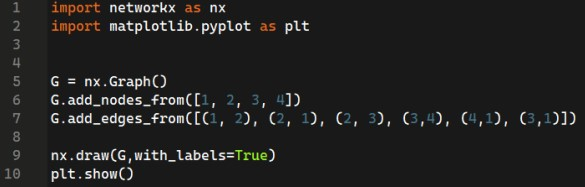`Answer :- d`

5. How many edges are there in the following graph?

• 4
• 5
• 3
• 2
`Answer :- a`

6. How many neighbors does node 3 have?

• 2
• 4
• 1
• 3
`Answer :- d`

7. In which of the following ways can we create a string in python?

• By using single quotes.
• By using double-quotes.
• By using triple-quotes.
• All of the above.
`Answer :- d`

8. Which of the following is not true about Stylometry Analysis?

• It is quantitative study of literature style
• It is based on the observation that the authors tend to write in relatively consistent and recognisable ways
• any two people may have same vocabulary
• It is a tool to study variety of questions involving style of writing
`Answer :- c`

9. A complete graph will have __ degree of separation

• 1
• 2
• 3
• Depends on the number of nodes.
`Answer :- a`

10. Networkx in pythons is used for

• Making networks
• Analyzing networks
• Visualizing networks
• All of the above
`Answer :- d`

## NPTEL The Joy Of Computing Using Python Week 8 Assignment Answer 2023

1. What is the correct initialisation of tuples?

Dates = [12,23,3,4]
Dates = (12,23,3,4)
Dates = {12,23,3,4}
Both B and C

`Answer :- b`

2. What operations can be done on tuples?

• Tuples are appendable.
• We can delete a value from tuples.
• Both A and B.
• We can count the number of instances of an element.
`Answer :- d`

3. What will be the output of the following code?

• 1,2,3,4,5
• 5,4,3,2,1
• 5,4,3,2
• 1,2,3,4
`Answer :- c`

4. What will be the output of the following code?

• gbdfcppl
• ezbdannj
• ytvxuhhd
`Answer :- a`

5. When the following program will clap?

• When both players will enter the same letters.
• When player 2 will enter the next letter with respect to player 1.
• When player 1 will enter the next letter with respect to player 2.
• It will never clap.
`Answer :- b`

6. Which statement is correct about the following program?

• The graph will go up when guess and pick are the same.
• The graph will go down when guess and pick are the same.
• The graph will go up when guess and pick are not the same.
• Both B and C
`Answer :- d`

7. What does NLTK do?

• Helps to work with human language data.
• Helps to convert machine data into human language.
• Helps to work on gibberish language.
• Helps to translate dog language into human language.
`Answer :- a`

8. What is the output of the following code?

• [‘!’, ‘e’, ‘e’, ‘e’, ‘h’, ‘h’, ‘r’, ‘t’, ‘y’]
• [‘h’, ‘e’, ‘y’, ‘!’, ‘t’, ‘h’, ‘e’, ‘r’, ‘e’]
• [‘y’, ‘t’, ‘r’, ‘h’, ‘h’, ‘e’, ‘e‘, ‘e’, ‘!’]
• None of the above
`Answer :- c`

9. While converting an image into black and white during enhancement you cannot convert it back into a colored image.

True
False

`Answer :- b`

10. How does vader help in sentiment analysis?

• It calculates whether the statement is negative, positive or neutral.
• It takes care of the intensity of a statement.
• Both A and B
• None of the above
`Answer :- c`

## NPTEL The Joy Of Computing Using Python Week 7 Assignment Answer 2023

1. Values of CSV files are separated by?

Commas
Colons
Semi-colons
Slash

`Answer :- a`

2. What is the output of the following code?

1, 2, 3, 7, 11, 10, 9, 5, 6
1, 2, 3, 5, 6, 7, 9, 10, 11
1, 5, 9, 10, 11, 7, 3, 2, 6
1, 5, 9, 2, 6, 10, 3, 7, 11

`Answer :- c`

3. What will be the output of the following code?

Scalar triangle
Right angle triangle
Equilateral triangle
Isosceles triangle

`Answer :- c`

4. Which of the following program will draw a hexagon?

`Answer :- b`

5. Which of the following library is used to render data on google maps?

gplot
gmplot
gmeplot

`Answer :- c`

6. What is the output of the following code?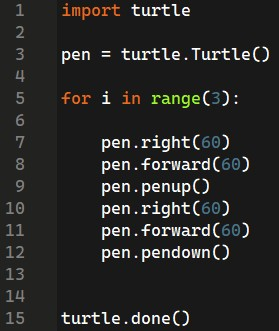`Answer :- c`

7. Which turtle command is equivalent to lifting up a pen.

penlift()
penup()
uppen()
penremove()

`Answer :- b`

8. Why do we use functions?

To reuse code blocks.
For the ease of code debugging.
All of the above

`Answer :- d`

9. Library used to import images?

PIL
Imageview
IMG
image

`Answer :- a`

10. In snakes and ladder what can be the ways to track ladders and snakes?

Maintain a dictionary with snakes or ladder number blocks as keys.
Using the if condition to check on every number.
Both A and B.
None of the above

`Answer :- c`

## NPTEL The Joy Of Computing Using Python Week 7 Programming Assignment Solution 2023

### Programming Assignment 1

Q. Given a sqaure matrix M, write a function DiagCalc which calculate the sum of left and right diagonals and print it respectively.(input will be handled by us)

Input:
A matrix M
[[1,2,3],[3,4,5],[6,7,8]]

Output
13
13

Explanation:
Sum of left diagonal is 1+4+8 = 13
Sum of right diagonal is 3+4+6 = 13

```Answer :-
def DiagCalc(M):
countL = 0
countR = 0
for i in range(len(M)):
countL += M[i][i]
countR += M[i][-1 - i]

print(countL)
print(countR)
n = int(input())
M = []
for i in range(n):
L = list(map(int, input().split()))
M.append(L)

DiagCalc(M)```

### Programming Assignment 2

Q. Given a matrix M write a function Transpose which accepts a matrix M and return the transpose of M.
Transpose of a matrix is a matrix in which each row is changed to a column or vice versa.

Input
A matrix M
[[1,2,3],[4,5,6],[7,8,9]]

Output
Transpose of M
[[1,4,7],[2,5,8],[3,6,9]]

```Answer :-
def Transpose(M):
rowlen = len(M)
collen = len(M)
ans  = [[] for i in range(collen)]
for i in range(collen):
for j in range(rowlen):
ans[i].append(M[j][i])
return ans
n = int(input())
M = []
for i in range(n):
L = list(map(int, input().split()))
M.append(L)
print(Transpose(M))```

### Programming Assignment 3

Q. Given a matrix M write a function snake that accepts a matrix M and returns a list which contain elements in snake pattern of matrix M. (See explanation to know what is snake pattern)

```Answer :- def snake(M):
rowlen = len(M)
collen = len(M)
ans  = []

for i in range(rowlen):
if i%2 == 0:
for j in M[i]:
ans.append(j)
else:
l = M[i]

for j in range(-1, -collen-1, -1):
ans.append(l[j])
return ans
n = int(input())
M = []
for i in range(n):
L = list(map(int, input().split()))
M.append(L)

print(snake(M))```

## NPTEL The Joy Of Computing Using Python Week 6 Assignment Answer 2023

1. What will be the output of the following code?

Shift every letter in a given word by value.
Shift every letter in a given word by 1.
Shift every letter in a given word by 26.
Returns the same word.

`Answer :- a`

2. In the list L = [4,6,7,4,6,2,1], What is the index of element ‘7’?

• 0
• 1
• 2
• 3
`Answer :- c`

3. Which of the following is true about recursion?

• Recursion always performs better than non-recursive code.
• Recursive code is easier to debug.
• The base case is necessary for recursion.
• Recursive code can be shorter than non-recursive code
`Answer :- b, c, d`

4. What will be the output of the following program?

• Calculating sum of first n terms.
• Calculating product of first n terms.
• Calculating power of first n terms.
• Calculating sum of last n terms.
`Answer :-  b`

5. In Caesar cipher,the mediator needs to make maximum of how many trails to break the code?

• 1
• 26
• no trail needed
• 10
`Answer :- b`

6. What is the output of the following program?

• 3628800
• Runs into an infinite loop
• 55
• Syntax error
`Answer :- b`

7. What’s the correct code for Binary search?

`Answer :- c`

8. Which of the following is TRUE about MIN-MAX strategy?

• Maximize the chances of your winning and minimize the changes of the opponent winning
• The game with min-max strategy can never be drawn
• Minimize the chances of your winning and maximize the chances of the opponent winning
• All the above are true
`Answer :- a`

9. A program that is written recursively cannot be written in a non-recursive manner.

• True
• False
`Answer :- b`

10. what will be the output of the following program?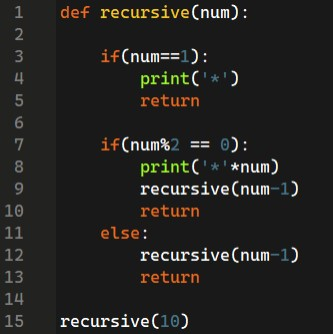**********
*********
********
*******
******
*****
****
***
**
*

*********
*******
*****
***
*

Runs into infinite loop

**********
********
******
****
**
*

```Answer :- d
**********
********
******
****
**
*```

## NPTEL The Joy Of Computing Using Python Week 6 Programming Assignment Solution 2023

### Programming Assignment 1

Question 1 : Aman likes to study about planets. Every night he goes outside and observe some planets with his telescope. Then he guesses the distance of each planet and pen it down. In this process he also pen down his favourite planet position. Given the distance of each planet to be unique you need to return position of Aman’s favourite planet after sorting all the distances.

Input : 5
[4,5,3,2,1]
2

Output : 5

``````N = int(input())
L = [int(i) for i in input().split()]
K = int(input())

favorite_distance = L[K - 1]
sorted_distances = sorted(L)
favorite_position = sorted_distances.index(favorite_distance) + 1

print(favorite_position, end="")``````

### Programming Assignment 2

Question 2 : Romeo and Juliet love each other. Romeo wants to send a message to Juliet and also don’t want anyone to read it without his permission. So he shifted every small letter in the sentence by -2 position and every capital letter by -3 position. (If the letter is c, after shifting to by -2 position it changes to a, and for D new letter will be A).
But the letter is too long and Romeo does not have enough time to encrypt his whole letter. Write a program to help Romeo which prints the encrypted message. You can assume there are no special characters except spaces and numeric value.

Input : Hello Juliet

Output : Ecjjm Gsjgcr

``````S = input()

def encrypt_message(message):
encrypted = ''

for char in message:
if char.islower():
encrypted_char = chr(((ord(char) - ord('a') - 2) % 26) + ord('a'))
elif char.isupper():
encrypted_char = chr(((ord(char) - ord('A') - 3) % 26) + ord('A'))
else:
encrypted_char = char
encrypted += encrypted_char

return encrypted

# Encrypt the message and print the result
encrypted_message = encrypt_message(S)
print(encrypted_message,end="")``````

### Programming Assignment 3

Question 3 : Write a function whole(N) which takes a number N and return the sum of first N whole number. Write the program using recursion.

Input : 3

Output : 6

``````def whole(N):
if N == 0:
return 0
else:
return N + whole(N - 1)

N = int(input())
print(whole(N))``````

## NPTEL The Joy Of Computing Using Python Week 5 Assignment Answer 2023

1. Which is the fastest sorting algorithm?

• Bubble Sort
• Bucket Sort
• Quick Sort
• Insertion Sort
`Answer :- c`

2. How can you remove all items from a dictionary?

• dict.clear()
• del dict
• dict.remove_all()
• dict.pop()
`Answer :- a`

3. What happens if you try to add a new key to a dictionary that already exists?

• The key and its associated value will be updated.
• The key and its associated value will be added.
• The key will be added, but the associated value will remain unchanged.
• An error will occur.
`Answer :- a`

4. Which of the following is true about dictionaries?

• There can be multiple same keys.
• Every value must be unique.
• Every key must be unique.
• We can’t get every key from the dictionary.
`Answer :- c`

5. What is the syntax to create a dictionary?

• D = []
• D = {}
• D = ()
• D = dictionary()
`Answer :- b`

6. What will be the output of the following code?

• ‘a’ ‘b’ ‘c’
• 20 30 50
• (‘a’, 20), (‘b’, 30), (‘c’, 50)
• (‘a’, ‘b’, ‘c’) (20, 30, 50)
`Answer :- c`

7. In the Monte Hall Problem, what is the probability of winning if you switch doors after the host reveals a goat behind one of the other doors?

• 1/3
• 1/2
• 2/3
• 3/4
`Answer :- c`

8. In the Monte Hall Problem, what is the probability of winning if you do not switch doors after the host reveals a goat behind one of the other doors?

• 1/3
• 1/2
• 2/3
• 3/4
`Answer :- a`

9. What is the name of the game show that the Monte Hall Problem was based on?

• Jeopardy
• Wheel of Fortune
• The Price is Right
• Let’s Make a Deal
`Answer :- d`

10. Which module in Python can be used for Speech to Text conversion?

• SpeechRecognition
• PyAudio
• Wave
• all of the above
`Answer :- a`

## NPTEL The Joy Of Computing Using Python Week 5: Programming Assignment Answer

Q1. You are given a string S. Write a function count_letters which will return a dictionary containing letters (including special character) in string S as keys and their count in string S as values.

(input and output will be handled by us you just need to write the function and return the dictionary)

Input
The Joy of computing

Output
{‘T’: 1, ‘h’: 1, ‘e’: 1, ‘ ‘: 3, ‘j’: 1, ‘o’: 3, ‘y’: 1, ‘f’: 1, ‘c’: 1, ‘m’: 1, ‘p’: 1, ‘u’: 1, ‘t’: 1, ‘i’: 1, ‘n’: 1, ‘g’: 1}

Explanation: T is appeared once in the string, similarly o is appeared 3 times in the string and so on. (You do not have to worry about the order of arrangement in your dictionary)

solution:-

```def count_letters(S):
ans = {}

for j in S:
if j in ans:
ans[j] += 1
else:
ans[j] = 1

return(ans)  ```

Q2. You are given a list L. Write a function uniqueE which will return a list of unique elements is the list L in sorted order. (Unique element means it should appear in list L only once.)

Input will be handled by us

Input
[1,2,3,3,4,4,2,5,6,7]

Output
[1,5,6,7]

Explanation

Elements 1,5,6,7 appears in the input list only once.

[ihc-hide-content ihc_mb_type=”show” ihc_mb_who=”1,2,3″ ihc_mb_template=”1″ ]

```def uniqueE(L):
ans=list()
for a in L:
if L.count(a)==1:
ans.append(a)
return(sorted(ans))   ```

Q3. You are given a list L. Write a program to print first prime number encountered in the list L.(Treat numbers below and equal to 1 as non prime)

Input will be handled by us.

Input
[1,2,3,4,5,6,7,8,9]

output
2

Explanation
Since 2 is the first prime number is list L, therefor it is printed.

```prime=[2, 3, 5, 7, 11, 13, 17, 19, 23, 29, 31, 37,
41, 43, 47, 53, 59, 61, 67, 71, 73, 79, 83, 89,
97, 101, 103, 107, 109, 113, 127, 131, 137, 139,
149, 151, 157, 163, 167, 173, 179, 181, 191, 193,
197, 199, 211, 223, 227, 229, 233, 239, 241, 251,
257, 263, 269, 271, 277, 281, 283, 293, 307, 311]
for j in L:
if j in prime:
print(j,end="")
break```

[/ihc-hide-content]

## NPTEL The Joy Of Computing Using Python Week 4 Assignment Answer 2023

1. What is a magic square?

A square grid of letters
A square grid of numbers where the sum of the rows, columns, & diagonals are equal
A special kind of card trick
A term used in cryptography

`Answer :- b`

2. In a 3×3 magic square, what is the magic constant?

• 3
• 6
• 9
• 15
`Answer :- d`

3. Which of the following is NOT a property of a magic square?

• The sum of each row is equal
• The sum of each column is equal
• The sum of each diagonal is equal
• The sum of each individual element is equal
`Answer :- d`

4. What will be the output of the following code?

• A magic square of size 2.
• A magic square of size n.
• A magic square of an even size.
• A magic square of an odd size.
`Answer :- b`

5. What will be the output of the following code?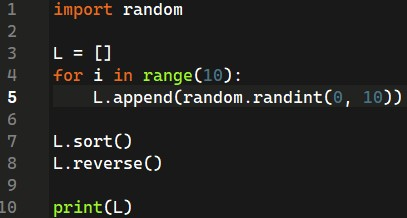• Sorted List(L) containing random elements between 0-10 in descending order.
• Sorted List containing random elements between 0-10 in ascending order.
• Sorted List containing elements between 0-10.
• Sorted List containing elements between 0-9 in ascending order.
`Answer :- a`

6. Which code will generate all prime numbers between 0-100?

`Answer :- b`

7. In the birthday paradox, as the number of people in a group increases, what happens to the probability that two people share a birthday?

• It increases
• It decreases
• It stays the same
• It becomes impossible
`Answer :- a`

8. Which module is used to generate random numbers in Python?

• math
• random
• stats
• numpy
`Answer :- b`

9. Which function is used to shuffle a list in Python?

• random.shuffle()
• shuffle()
• list.shuffle()
• random_list()
`Answer :- a`

10. What is the output of the following code?

import random
nums = [1, 2, 3, 4, 5]
random.shuffle(nums)
print(nums)

• [1, 2, 3, 4, 5]
• [5, 4, 3, 2, 1]
• A random ordering of the numbers 1 through 5
• An error
`Answer :- c`

## NPTEL The Joy Of Computing Using Python Week 3 Assignment Answer 2023

1. What will be the output of the following code?

L = [‘a’, ‘b’, ‘c’, ‘d’, ‘e’, ‘f’, ‘g’, ‘h’]
print(L[2:5])

• a, b, c
• a, b, c, d
• c, d, e
• c, d, e, f
`Answer :- c`

2. Which of the following is a valid way to declare a dictionary in Python?

• {1: “one”, 2: “two”, 3: “three”}
• [1: “one”, 2: “two”, 3: “three”]
• (1: “one”, 2: “two”, 3: “three”)
• <1: “one”, 2: “two”, 3: “three”>
`Answer :- a`

3. Which of the following method is correct to add an element at a specific position?

• insert()
• append()
• index()
`Answer :- a`

4. What is the correct syntax to add an item to the end of a list in Python?

• list.append(item)
• list.insert(item)
• list.extend(item)
`Answer :- b`

5. Which of the following is not a valid data type in Python?

• integer
• string
• float
• character
`Answer :- d`

6. What is the output of the following code?

``````for i in range(1, 21):
if i % 3 == 0 and i % 5 == 0:
print("FizzBuzz")
elif i % 3 == 0:
print("Fizz")
elif i % 5 == 0:
print("Buzz")
else:
print(i)``````
• Prints numbers from 1 to 20
• Prints Fizz for multiples of 3 and Buzz for multiples of 5
• Prints FizzBuzz for multiples of 3 and 5
• None of the above
`Answer :- b`

7. What is the output of the following code?

a = 5
b = 2
print(a // b)

• 2
• 2.5
• 3
• 2.0
`Answer :- a`

8. What is the output of the following code?
s = “hello”
print(s[::-1])

• “hello”
• “olleh”
• “hlo”
• “leh”
`Answer :- b`

9. What is the output of the following code?
a = 10
b = 5
c = a % b
print(c)

• 2
• 5
• 0
• 1
`Answer :- c`

10. What is the output of the following code?
s = “python”
print(s[1:4])

• “pyt”
• “yth”
• “tho”
• “hon”
`Answer :- b`

## NPTEL The Joy Of Computing Using Python Week 2 Assignment Answer 2023

1. Which of the following is a high-level programming language?

• Assembly
• C
• Python
• Machine Language
`Answer :- c`

2. Which of the following is an example of a front-end programming language?

• PHP
• Java
• HTML
• SQL
`Answer :- c`

3. Which of the following is used to store data in a programming language?

• Variables
• Arrays
• Loops
• Conditionals
`Answer :- a, b`

4. Which statement will print ‘The joy of computing’?

• print(The joy of computing)
• print The joy of computing
• printf(‘The joy of computing)
• print(‘The joy of computing’)
`Answer :- d`

5. What should be the value of _ to print all numbers from 0-10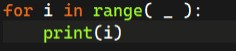• 10
• 9
• 11
• None of the above
`Answer :- c`

6. Which of the following keyword is used to declare a function in a programming language?

• def
• var
• int
• float
`Answer :- a`

7. What will be the output of the following code?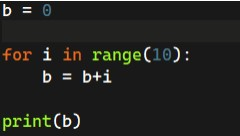• 0
• 45
• 43
• 50
`Answer :- b`

8. What value will c store in it after the execution of the below code?
c=b**3

• Value of b multiplied by 3
• Cube of b
• Value of b multiplied with 3 twice
• It will throw an error
`Answer :- d`

9. What will be the output? suppose the input is 30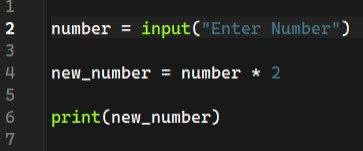• 60
• Error
• 3030
• 30
`Answer :- c`

10. What is the output for the following program?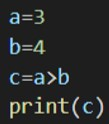• True
• False
• It will display an error message
• Boolean
`Answer :- b`

## NPTEL The Joy Of Computing Using Python Week 1 Assignment Answer 2023

1. What will be the value of my variable at the end of the loop.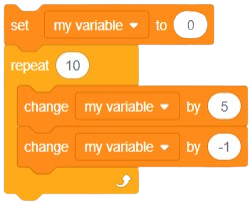• 50
• 40
• 49
• 0
`Answer :- 40`

2. Which of the following is not a type of block in Scratch?

• Motion
• Looks
• Sound
• Jump
`Answer :- Jump`

3. What is the main function of the “if” block in Scratch?

• To repeat a set of instructions
• To create a loop
• To control the flow of the program based on a condition
• To play a sound
`Answer :- To control the flow of the program based on a condition`

4. Which block in Scratch is used to control the movement of a sprite?

• Motion
• Looks
• Sound
• Control
`Answer :- Motion`

5. Imagine sprite to be a scooter. How many times scooter will move forward?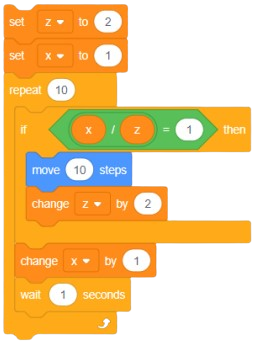• 7
• 4
• 6
• 5
`Answer :- 5`

6. Which block in Scratch is used to make a sprite say something?

Motion
Looks
Sound
Control

`Answer :- Looks`

7. What is the purpose of the “repeat” block in Scratch?

• To repeat a set of instructions a specific number of times
• To repeat a set of instructions forever
• To repeat a set of instructions based on a condition
• To play a sound repeatedly
`Answer :- To repeat a set of instructions a specific number of times`

8. Which block in Scratch is used to wait for a certain amount of time before continuing with the program?

• Motion
• Looks
• Sound
• Control
`Answer :- Control`

9. Which block in Scratch is used to detect when a sprite touches another sprite?

• Motion
• Looks
• Sound
• Sensing
`Answer :- Sensing`

10. What is the purpose of the “broadcast” block in Scratch?

• To send a message to another sprite
• To play a sound
• To move a sprite
• To change the background color
`Answer :- To send a message to another sprite`
Scroll to Top